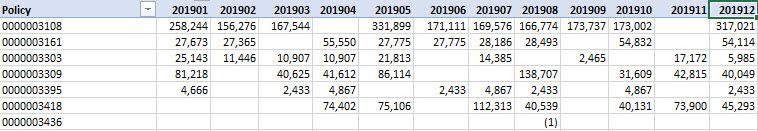cancel
Showing results for
Did you mean:

Fabric is Generally Available. Browse Fabric Presentations. Work towards your Fabric certification with the Cloud Skills Challenge.Helper I

## Difference from previous period (in YYYYMM format)

Hello,

I have a column named PERIOD (in YYYYMM) format in both the fact and calendar tables.

Is there a way to calculate the difference between current period and previous period in the pivot?

Difference between 201902 and 201901 should be 156,276 - 258,244 = (101968) and so on. I want to show the actual values and difference in columns (pivoted format).1 ACCEPTED SOLUTIONSuper User

@chandakaushik , better is that you create a date , as suggested by @lbendlin, and use a date calendar.

And create measures like this

``````MTD Sales = CALCULATE(SUM(Sales[Sales Amount]),DATESMTD('Date'[Date]))
last MTD Sales = CALCULATE(SUM(Sales[Sales Amount]),DATESMTD(dateadd('Date'[Date],-1,MONTH)))
last month Sales = CALCULATE(SUM(Sales[Sales Amount]),previousmonth('Date'[Date]))
last MTD (complete) Sales =  CALCULATE(SUM(Sales[Sales Amount]),DATESMTD(ENDOFMONTH(dateadd('Date'[Date],-1,MONTH))))
previous month value =  CALCULATE(sum(''Table''[total hours value]),previousmonth('Date'[Date]))

diff = [MTD Sales]-[last MTD Sales]
diff % = divide([MTD Sales]-[last MTD Sales],[last MTD Sales])
``````

The second way is to have month year table (Your month are sortable) and create a rank. You need to have a separate table.

``````Month Rank = RANKX(all('Date'),'Date'[Month],,ASC,Dense)
This Month = CALCULATE(sum('order'[Qty]), FILTER(ALL('Date'),'Date'[Month Rank]=max('Date'[Month Rank])))
Last Month = CALCULATE(sum('order'[Qty]), FILTER(ALL('Date'),'Date'[Month Rank]=max('Date'[Month Rank])-1))``````

To get the best of the time intelligence function. Make sure you have a date calendar and it has been marked as the date in model view. Also, join it with the date column of your fact/s. Refer :

3 REPLIES 3Super User

@chandakaushik , better is that you create a date , as suggested by @lbendlin, and use a date calendar.

And create measures like this

``````MTD Sales = CALCULATE(SUM(Sales[Sales Amount]),DATESMTD('Date'[Date]))
last MTD Sales = CALCULATE(SUM(Sales[Sales Amount]),DATESMTD(dateadd('Date'[Date],-1,MONTH)))
last month Sales = CALCULATE(SUM(Sales[Sales Amount]),previousmonth('Date'[Date]))
last MTD (complete) Sales =  CALCULATE(SUM(Sales[Sales Amount]),DATESMTD(ENDOFMONTH(dateadd('Date'[Date],-1,MONTH))))
previous month value =  CALCULATE(sum(''Table''[total hours value]),previousmonth('Date'[Date]))

diff = [MTD Sales]-[last MTD Sales]
diff % = divide([MTD Sales]-[last MTD Sales],[last MTD Sales])
``````

The second way is to have month year table (Your month are sortable) and create a rank. You need to have a separate table.

``````Month Rank = RANKX(all('Date'),'Date'[Month],,ASC,Dense)
This Month = CALCULATE(sum('order'[Qty]), FILTER(ALL('Date'),'Date'[Month Rank]=max('Date'[Month Rank])))
Last Month = CALCULATE(sum('order'[Qty]), FILTER(ALL('Date'),'Date'[Month Rank]=max('Date'[Month Rank])-1))``````

To get the best of the time intelligence function. Make sure you have a date calendar and it has been marked as the date in model view. Also, join it with the date column of your fact/s. Refer :Helper I

Thank you guys, @amitchandak @lbendlin ! Appreciate your guidence and help!Super User

Convert the period to a fake date

DATE(YYYY,MM,1)

and then tie that date into the calendar table. From there you can then use the regular time intelligence functions.Announcements#### Power BI Monthly Update - November 2023

Check out the November 2023 Power BI update to learn about new features.#### The largest Power BI and Fabric virtual conference

130+ sessions, 130+ speakers, Product managers, MVPs, and experts. All about Power BI and Fabric. Attend online or watch the recordings.Top Solution Authors
Top Kudoed Authors
Users online (5,932)